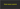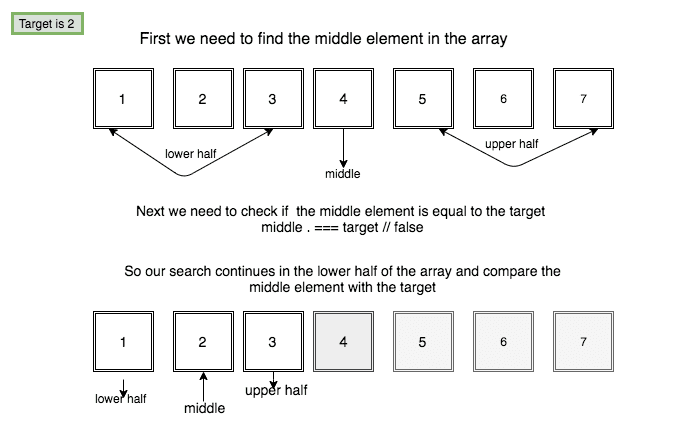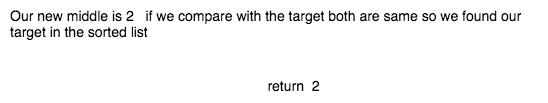# How to implement a Binary search algorithm in JavaScriptIn this tutorial, we are going to learn about binary search algorithm and its implementation in JavaScript.

In computer science, the binary search algorithm is used to find the position of the target value in a sorted array.

## How binary search algorithm works?

Binary search algorithm compares the target value to the middle element in the array. if the target is found then return the index or search continues in the other half of the array repeating until a target is found.

Let’s learn by using a sample sorted array.## Binary search algorithm implementation

Pseudocode

1. Store the midpoint of the array.
2. compare the midpoint to the target value.

• return item
3. If midpoint is less than the target value then search continues in the upper half of the array.
4. If midpoint is greater than the target value then search continues in the lower half of the array.

we are using the recursive approach.

``````function binarySearch(arr, target) {

// calculating midpoint
const midpoint = Math.floor(arr.length / 2);

// if the target is equals to midpoint then search is found
if (arr[midpoint] === target) {
return arr[midpoint];
}
// search continues upperpart of the array
if (arr[midpoint] < target && arr.length > 1) {
return binarySearch(arr.slice(midpoint), target);
}
// search continues lowerpart of the array
if (arr[midpoint] > target && arr.length > 1) {
return binarySearch(arr.slice(0, midpoint), target);
}

return false;
}

console.log(binarySearch([1, 2, 3, 4, 5, 6, 7, 8, 9, 10, 11], 10));

// output 10``````

Time complexity : O(logN).

## Tests

Hope you enjoyed…

## Top Udemy Courses##### JavaScript - The Complete Guide 2020 (Beginner + Advanced)
45,614 students enrolled
52 hours of video content
View Course##### React - The Complete Guide (incl Hooks, React Router, Redux)
284,472 students enrolled
40 hours of video content
View Course##### Vue - The Complete Guide (w/ Router, Vuex, Composition API)
130,921 students enrolled
48.5 hours of video content
View Course